## 【DP】String Distance – 20HDU多校D2T12

### 传送门

|q|\leq10^5次询问，每次给出n的一个区间[l,r]

f[i][j]表示B[i]为止的，长度为jLCSA串的最短前缀长度。

f[i][j]=min(f[i-1][j],g[l+f[i-1][j-1]][b[i]]-l+1)

#include<bits/stdc++.h>
using namespace std;
const int maxn = 100010;
int e[maxn], f;
char s[maxn], p;
int main()
{
freopen("1012.in", "r", stdin);
freopen("r.txt", "w", stdout);
cin.tie(0); cout.tie(0); ios::sync_with_stdio(0);
int T; cin >> T;
while(T--)
{
cin >> (s + 1) >> (p + 1);


## 【数论】Fibonacci Sum – 20HDU多校1-5

### 传送门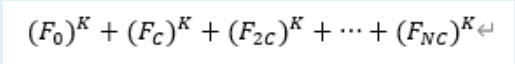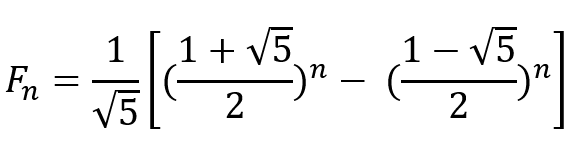383008016^2 ≡616991993^2≡5 (mod 10^9+9)

F_n=C(A^n-B^n)

F_{cn}^k=C^k(A^{cn}-B^{cn})^k

\sum\limits_{i=0}^n F_{ci}^k=C^k\sum\limits_{i=0}^n(A^{ci}-B^{ci})^k

\sum\limits_{i=0}^nF_{ci}^k= C^k\sum\limits_{i=0}^n \sum\limits_{r=0}^k \binom{k}{r}(A^{ci(k-r)}B^{cir}(-1)^r)

\sum\limits_{i=0}^nF_{ci}^k= C^k \sum\limits_{r=0}^k \binom{k}{r} (-1)^r \sum\limits_{i=0}^n (A^{ci(k-r)}B^{cir})• 注意本题从0累加到n实际上有n + 1项，求几何级数的时候需要注意。但是第0项等于0，所以也可以看作从1开始枚举，用通项的时候单独减去1。两种处理方法都可以。
• 第二个需要注意的点是公式仅适用于r =\not 1。如果r=1直接用r * n或者r*(n+1)即可。取决于上面一步是什么处理方法。

#pragma GCC optimize(2)
#include<bits/stdc++.h>
using namespace std;
typedef long long LL;
const int mod = 

## 转送门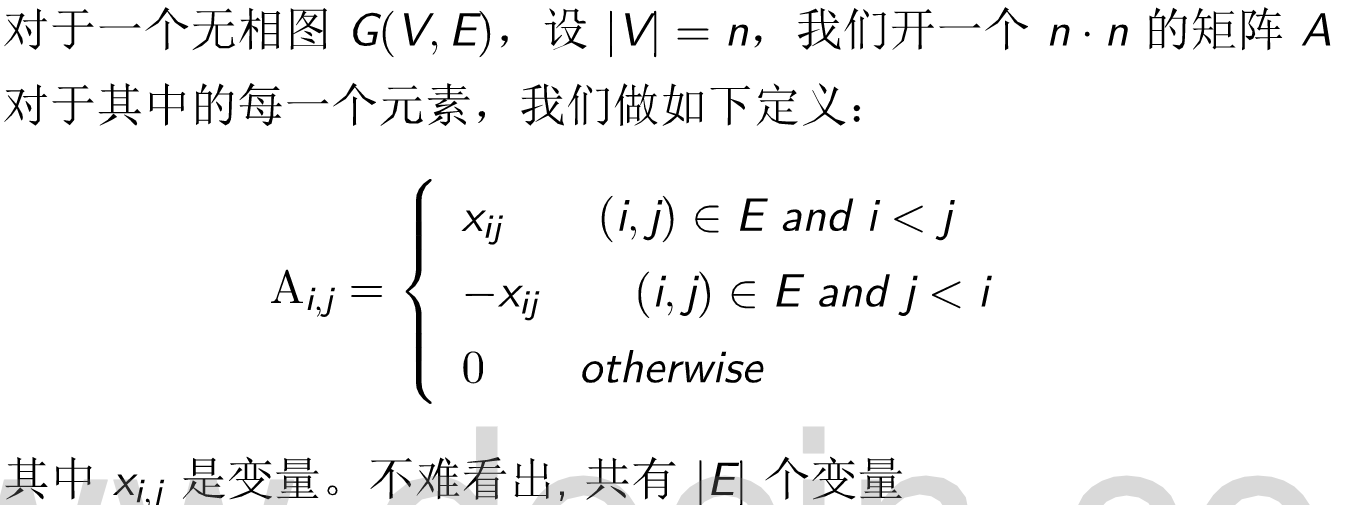#include<bits/stdc++.h>
using namespace std;
typedef long long ll;
mt19937 mrand(random_device{}()); // mrand是给定随机数种子的的大随机数对象
int rnd(int x) { return mrand() % x;}
const double eps = 1e-6;
const int maxn = 433;
ll a[maxn][maxn];
ll mod = 1e9 + 7;
ll ksm(ll n, ll k)
{
ll res = 1;
while(k)
{
if(k & 1) res = res * n % mod;
n = n * n % mod;
k >>= 1;
}
return res;


## 传送门

#include<bits/stdc++.h>
using namespace std;
const int maxn = (1 << 18) + 233;
typedef long long ll;
const ll mod = 1e9 + 7;
ll inv = 0;
ll ksm(ll n, ll k)
{
ll res = 1;
while(k)
{
if(k & 1) res = res * 

## 原题传送门

### 中文题面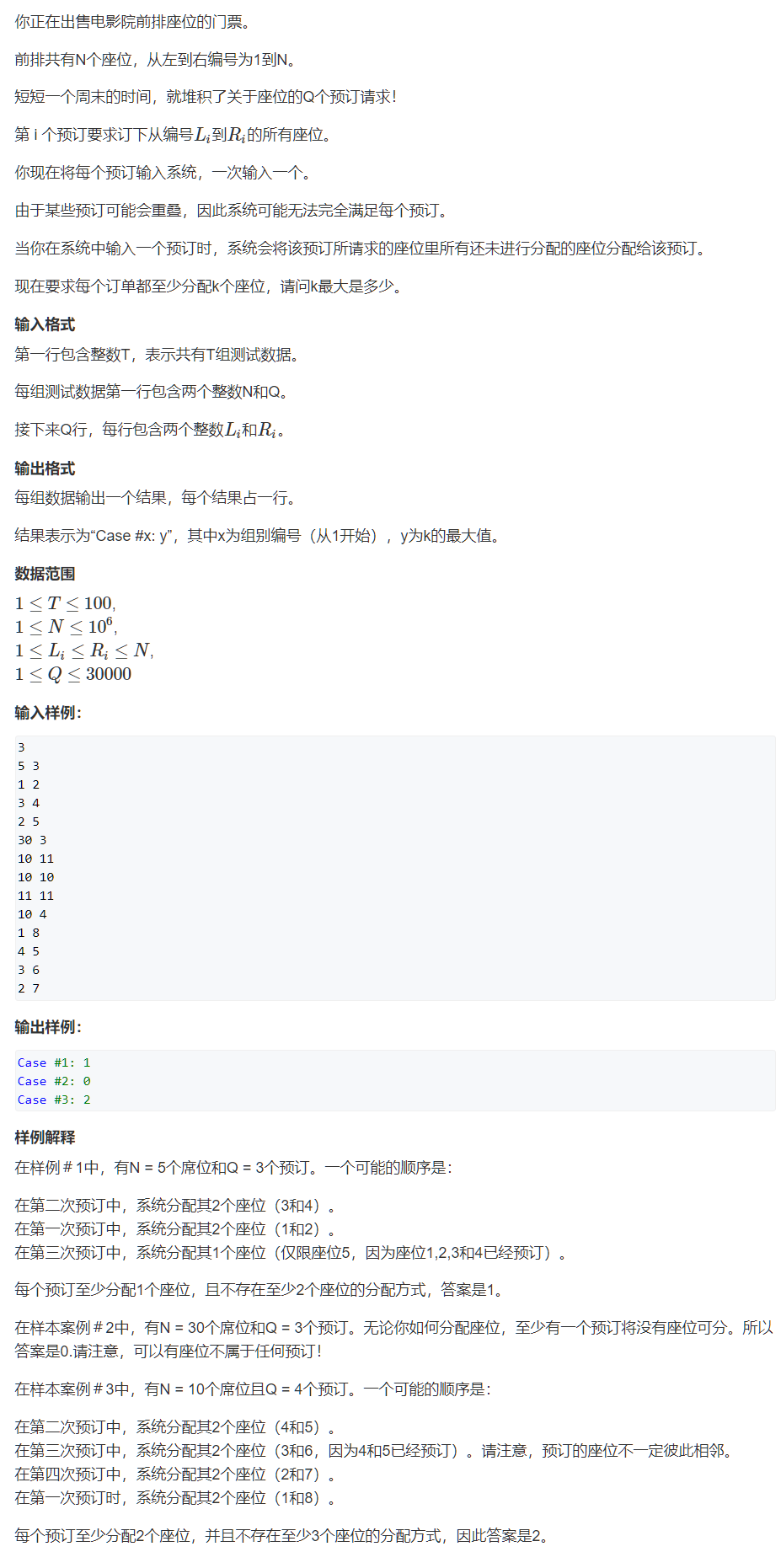1. 从最后一个人开始，枚举谁能得到最多的座位。假设是第X个询问。
2. 去掉第X个询问，再重复步骤一。
3. 重复以上步骤直到询问为空。从得到的答案里取一个最小值即是答案。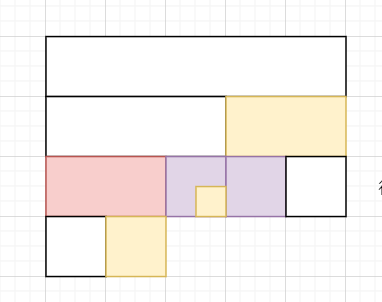#include<cstdio>
#include<algorithm>
#include<unordered_set>
#include<iostream>
#include<cstring>
using namespace std;
typedef pair<int, int> PII;
const int maxn = 1e6 + 23;
const int INF = 0x3f3f3f3f;
int n, q;
PII seg;
struct NodeQ {
int l, r;
int v;
}trQ[30300 * 4];
void buildQ(int u, int l, int r)
{
if (l == r) 

## 传送门

N和K都小于1E6

f[i][j] 表示前 i 天偷了 j 天懒。也就是说本来应该走i * k 个景点，但是只走了i * k – j个。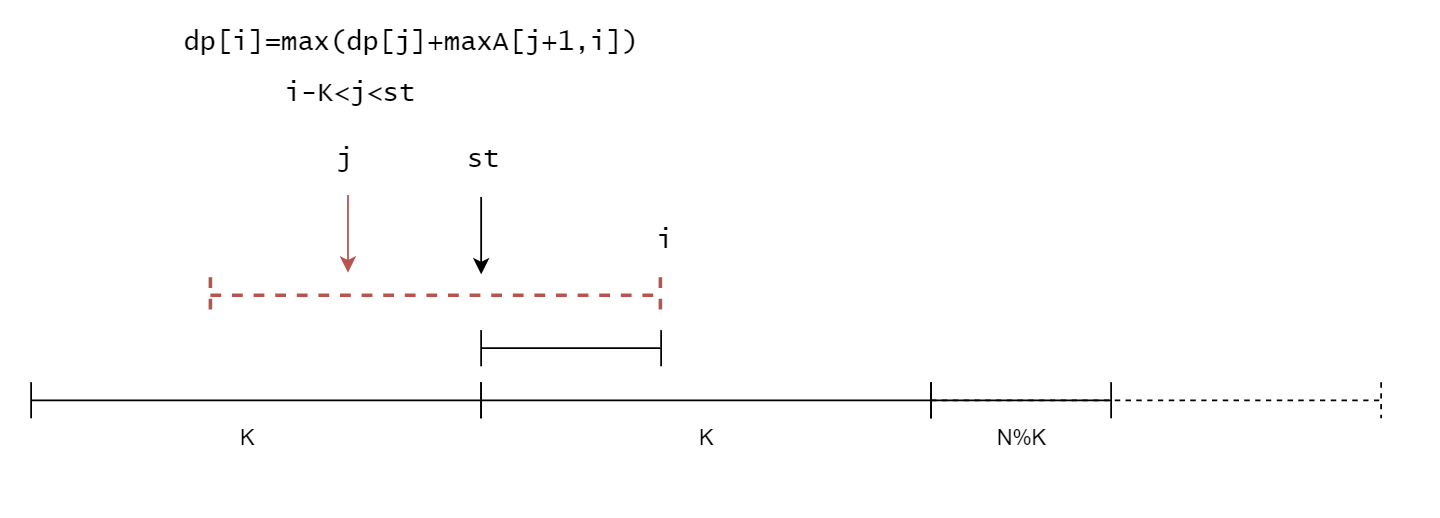#include<bits/stdc++.h>
using namespace std;
typedef long long ll;
const int maxn = 1e6 + 233;
ll c[maxn], 

## Z-algorithm(Z函数)模板

#include<bits/stdc++.h>
using namespace std;
const int maxn = 1e6 + 233;
char str[maxn];
int ne[maxn], z[maxn];
int main()
{
int T; scanf("%d", &T);
while(T--)
{
scanf("%s", str + 1);
int len = strlen(str + 1);
for(int i = 1; i <= len; i++) z[i] = 0;
z = len;
for(int i = 2, l = 0, r = 0; i <= len; i++)
{
if(i <= r) z[i] = min(z[i - l + 1], r - 

## 【数论】一道神奇的数论题

A知道其乘积，B知道其和。

A：我不知道这两个数，但我确定B也不知道
B：我也不知道这两个数，但我确定A也不知道
A：我知道了
B：我知道了



1. A第一句话首先表明了t肯定有大于一种合法的分解方法。
2. A的第二句话说“B不知道”，说明了s \in [7,195]，因为如果s=5则只能为2+3，其他数同理。
3. B的第一句话证明了A第二句话的是正确的。
4. B的第二句话确定“A不知道”，说明s=x+y无论写成什么形式，t=xy都不可能只有一种分解方法，也就是说s肯定不是两个质数的和。

11 17 23 27 29 35 37 41 47 51 53 57 59 65 67 71 77 79 83 87 89 93 95 97 101 103 105 107 109 111 113 115 117 119 121 123 125 127 129 131 133 135 137 139 141 143 145 147 149 151 153 155 157 159 161 Read the rest

## 【线段树】区间不重复覆盖

### 传送门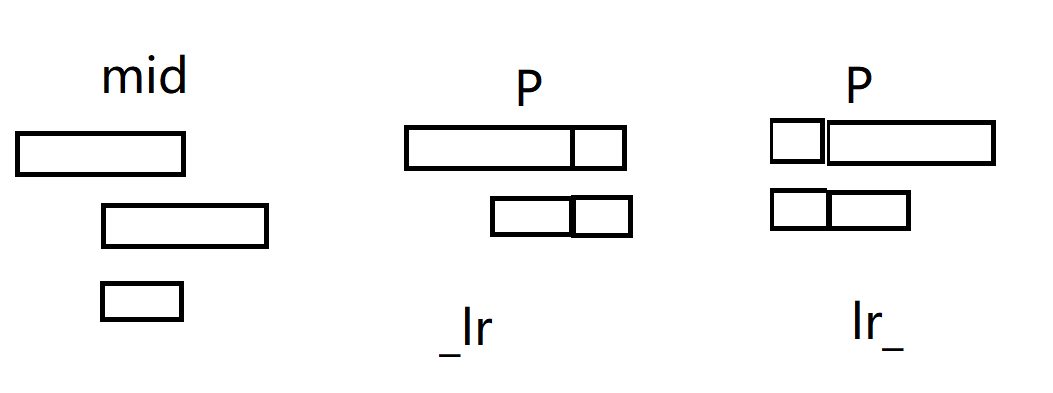“_LR” 代表区间左端点可以填为长度为1或者2的右端点，但右端点不超出。即可能向左超出
“LR_” 代表区间右端点可以填为长度为1或者2的左端点，但左端点不超出。即可能向右超出
“_LR_” 代表左右端点都有可能超出。
“L_R” 代表左右端点都不超出。

#include<bits/stdc++.h>
using namespace std;
const int maxn = 1e5 + 23;
struct Node{
int l, r;
int s, sl, sr, sn, lv, rv;
}tr[maxn * 4];
void pushup(Node &u, Node &l, Node &r)
{
u.lv = l.lv;
u.rv = r.rv;
int mv = l.rv & (1 << 1);
u.s = (l.s && r.s) || (l.sl && r.sr && mv);
u.sl = (l.s && r.sl) || (l.sl 

# 股票问题汇总

1. 不能进行多笔交易；买股票之前只能先把手上的股票卖出。一般此类题是dp。
2. 可以进行多笔交易；手里可以同时持有多只股票。一般要贪心。

#include<bits/stdc++.h>
using namespace std;
const int maxn = 1e5 + 233;
int w[maxn], f[maxn];
int main()
{
int n, k; scanf("%d%d", &n, &k);
for(int i = 1; i <= n; i++) scanf("%d", &w[i]);
memset(f, 0xcf, sizeof f);
for(int i = 0; i <= n; i++) f[i] = 0;
for(int i = 1; i <= n; i++)
{
for(int j = 1; j <= k; j++)
{
f[i][j] = max(f[i -